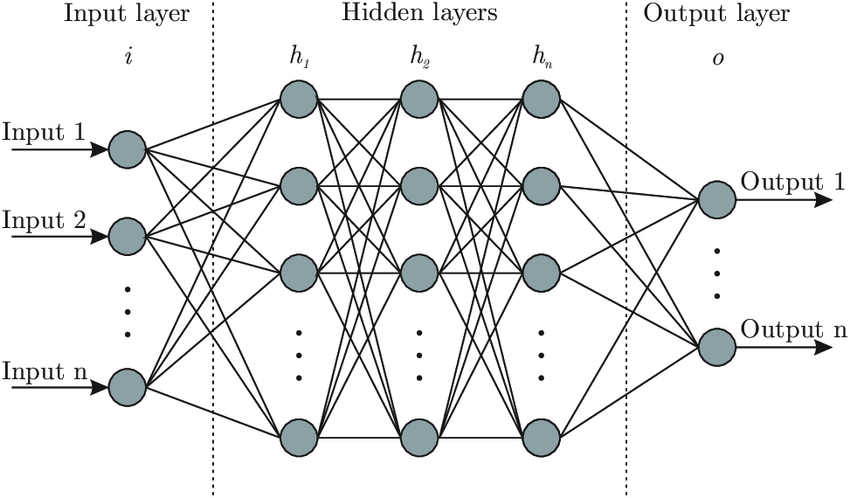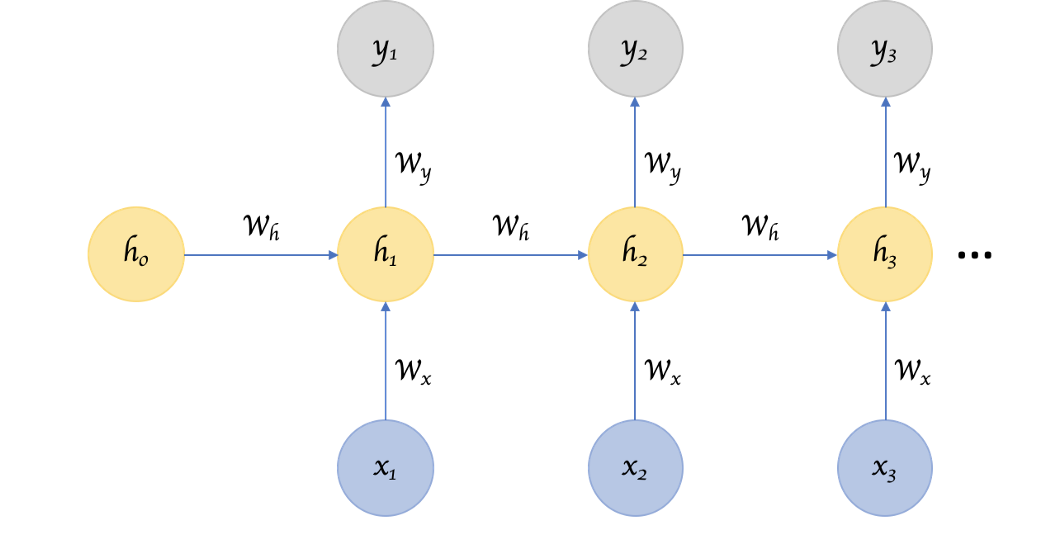# From Basics to Detail, Know Everything About Machine Learning

By NIIT Editorial

Published on 22/10/2021

8 minutes

Machine Learning was introduced in 1959, by an American pioneer in the field of computer gaming and artificial intelligence named Arthur Samuel. He said “it gives computers the ability to learn without being explicitly programmed”.

Machine learning (ML) is the study of computer algorithms that enhance on their own through experience and by the use of data. It is a part of artificial intelligence (AI). Machine learning algorithms construct a model established on sample data, known as "training data", to make assumptions or decisions without being instructed. Machine learning algorithms are used in a vast variety of applications, such as email filtering and computer vision.

Introduction to Deep Learning

Deep learning is a function of artificial intelligence (AI) that emulates the workings of the human brain in refining data and establishing patterns to use in decision making. Deep learning is a part of machine learning in artificial intelligence. It is also recognized as a deep neural learning or deep neural network. Deep learning AI is capable of learning without human administration, drawing from data that is both unstructured and unlabelled. It is also useful to detect fraud or money laundering, among other functions.

Deep learning vs Machine learning

In practical terms, deep learning is just a subdivision of machine learning but its capabilities are distinct. Machine learning uses algorithms to divide data and learn from that data it also makes informed decisions based on what is to be learned. Deep learning arranges algorithms in layers to build an "artificial neural network” that can learn and make intelligent decisions with commands.

 S.No. Machine Learning Deep Learning 1. Machine Learning is considered as a superset of Deep Learning Deep Learning is a subcategory of Machine Learning 2. The data represented in Machine Learning uses structured data. The data representation in Deep Learning uses neural networks (ANN). 3. Machine Learning is a transition of AI Deep Learning is a transition to Machine Learning. 4. Machine learning contains data points in thousands. Deep learning consists of millions of data points. 5. Outputs of Machine Learning is any numerical Value, like the classification of score Output in Deep learning is anything from numerical values to free-form elements. 6. It uses several types of automatic algorithms that turn to classify functions and predict future actions from the data. Deep learning neural network that approves data through processing layers to interpret features of data and their relations. 7. Algorithms are detected by data analysts to evaluate particular variables in data sets. Algorithms are largely self- illustrated on data analysis once settled into production. 8. Machine Learning is used to compete and learn new things. Deep Learning figures out complicated machine learning issues.

The different types of neural networks in deep learning are convolutional neural networks (CNN), recurrent neural networks (RNN), artificial neural networks (ANN), which change the way we interact with the world. These different types of neural networks are at the core of the deep learning revolution.

1. Artificial Neural Network (ANN)

A single perceptron (or neuron) can be comprehended as a Logistic Regression. An Artificial Neural Network (ANN), is a group of multiple perceptrons/ neurons at each layer. ANN is also called a Feed-Forward Neural network because inputs are processed only in the forward direction. It comprises 3 layers – Input, Hidden and Output. The input layer receives the inputs, the hidden layer processes the inputs, and the output layer generates the result.

ANN is being used around the industries such as Healthcare, Finance, Meteorology and Learning Management systems. It has a vast scope for individuals with knowledge in Artificial Neural Networks.

Pros

• Artificial Neural Network is eligible for learning any nonlinear function. These networks are popularly known as Universal Function Approximators.

• Neural networks are so flexible that they can be used for both regression and classification problems. The neural network is a mathematical model with approximation functions therefore any data which can be made numeric can be used in the model.

• The predictions are very fast, when trained.

• Neural networks work adequately with more data points.

Cons

• Neural networks are black boxes, which means we cannot know how much each dependent variable is influenced by the independent variable.

• It is computationally very costly and time-consuming to educate with traditional CPUs.

• Problems like overfitting and generalization occur frequently as neural networks depend a lot on training data.Source: Research Gate

2. Convolutional neural networks (CNN)

The name “convolutional neural network” demonstrates that the network engages a mathematical operation called convolution. Convolutional networks are specialized type of neural networks because they use convolution instead of common matrix multiplication in at least one of their layers.

Pros

• CNN learns the filters automatically, these filters help in taking out the right and appropriate features from the input data.

• CNN captures the spatial features from an image, which helps in observing the object accurately, the location of an object, as well as its relation with other objects in an image.

• In CNN, a single filter is pertained across various parts of input to produce a feature map as it follows the concept of parameter sharing.

Cons

• A convolutional neural network is considerably slower when compared to others due to operations like maxpool.

• If the computer doesn’t contain a good GPU then CNN takes a lot of time in the training process.

• It requires a large Dataset to process and train the neural network.

3. Recurrent Neural Network (RNN)

A recurrent neural network (RNN) is a section of artificial neural networks where a directed graph is formed along a secular sequence between the connection of nodes. RNNs can use their internal memory to process variable length progressions of inputs.

The term “recurrent neural network” is referred to as two large classes of networks with a related general structure. The first is finite impulse and the other is infinite impulse. Both of these classes of networks display temporal dynamic behaviour. A finite impulse recurrent network is a directed acyclic graph whereas an infinite impulse recurrent network is a directed cyclic graph that cannot be unrolled.

Pros

• An RNN remembers every information given through time, which helps in time series prediction. This process is called Long Short Term Memory.

• Recurrent neural networks are even used with convolutional layers to broaden the effective pixel JC neighbourhood.

Cons

• Highly disappearing and exploding problems.

• Training an RNN is a very tough job.

• It cannot function in very long sequences.Source: towards data science

CNN vs RNN vs ANN

 ANN CNN RNN Type of data Data is found in tabular and text form Data is found in image form. Data is arranged in sequence form. Parameter sharing No Yes Yes Fixed length input Yes Yes No Recurrent connections No No Yes Disappearing and exploding rise Yes Yes Yes Performance ANN is looked as less powerful when compared with the other two. CNN is considered as more capable than both RNN and ANN. RNN contains lesser compatible features than CNN. Applications Face recognition and computer vision. Natural language processing, digitalization of the text and face recognition. Converting text-to-speech

Want to learn data science, enrol in NIIT

NIIT’s Advanced Post Graduate Program in Data Science & Machine Learning is designed for learners who want to deep-skill in subjects like machine learning, deep learning, natural language processing, predictive modeling, and visualization to name some. This 18-week full time program offers placement assurance with a minimum CTC of Rs.5LPA (Terms & Conditions Apply). Job offers vary from profiles of a data analyst to business analyst.

Apply now!

### Advanced PGP in Data Science and Machine Learning (Full-Time)

Be job-ready! Earn a min. CTC of ₹8LPA with this placement-assured program*

Placement Assured Program*

Practitioner Designed